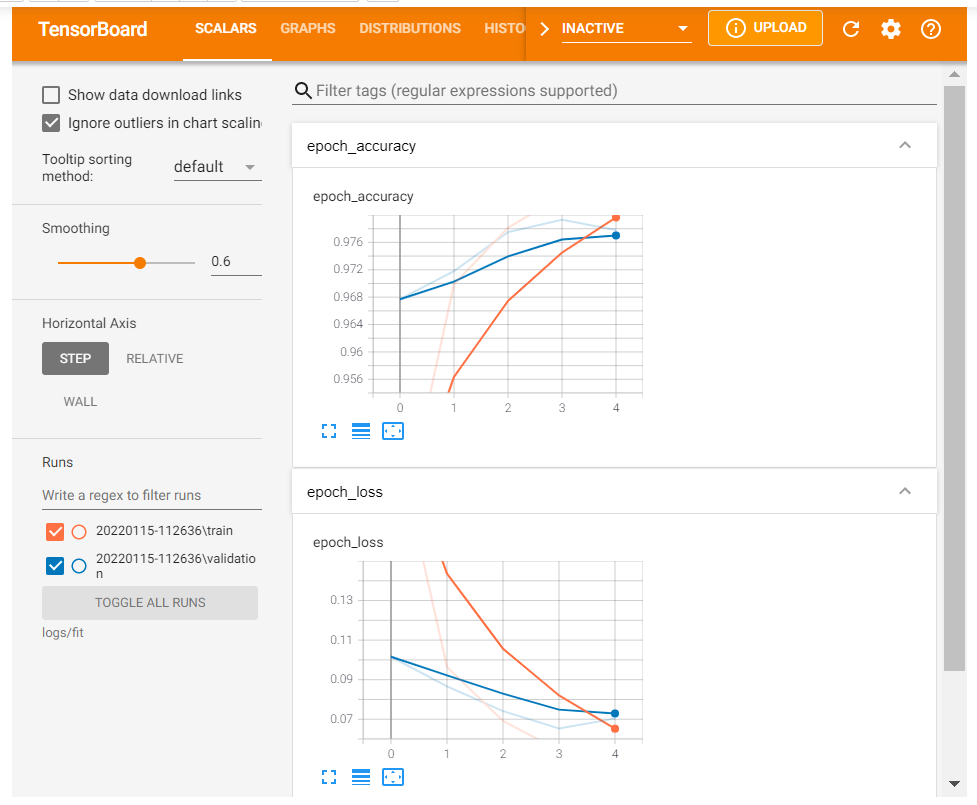top of page
Search

# Get started with TensorBoard In Machine Learning

In machine learning, to improve something you often need to be able to measure it. TensorBoard is a tool for providing the measurements and visualizations needed during the machine learning workflow. It enables tracking experiment metrics like loss and accuracy, visualizing the model graph, projecting embeddings to a lower dimensional space, and much more.

This quickstart will show how to quickly get started with TensorBoard. The remaining guides in this website provide more details on specific capabilities, many of which are not included here.

```# !pip install -q tf-nightly-2.0-preview
# if you want to use the tf2.0 please uncomment the above line
# Load the TensorBoard notebook extension

# there are other ways of doing this: https://www.dlology.com/blog/quick-guide-to-run-tensorboard-in-google-colab/ you can try this way also
```import tensorflow as tf
import datetime```

Using the MNIST dataset as the example, normalize the data and write a function that creates a simple Keras model for classifying the images into 10 classes.

```mnist = tf.keras.datasets.mnist

x_train, x_test = x_train / 255.0, x_test / 255.0

def create_model():
return tf.keras.models.Sequential([
tf.keras.layers.Flatten(input_shape=(28, 28)),
tf.keras.layers.Dense(512, activation='relu'),
tf.keras.layers.Dropout(0.2),
tf.keras.layers.Dense(10, activation='softmax')
])```

### Using TensorBoard with Keras Model.fit()

When training with Keras's Model.fit(), adding the TensorBoard callback will ensure logs are created and stored. Additionally, enable histogram computation every epoch with histogram_freq=1 (this is off by default)

Place the logs in a timestamped subdirectory to allow easy selection of different training runs.

```model = create_model()
loss='sparse_categorical_crossentropy',
metrics=['accuracy'])

log_dir="logs/fit/" + datetime.datetime.now().strftime("%Y%m%d-%H%M%S")

model.fit(x=x_train,
y=y_train,
epochs=5,
validation_data=(x_test, y_test),
callbacks=[tensorboard_callback])

```

Output:Start TensorBoard through the command line or within a notebook experience. The two interfaces are generally the same. In notebooks, use the %tensorboard line magic. On the command line, run the same command without "%".

`%tensorboard --logdir logs/fit`

Output:A brief overview of the dashboards shown (tabs in top navigation bar):

• The Scalars dashboard shows how the loss and metrics change with every epoch. You can use it to also track training speed, learning rate, and other scalar values.

• The Graphs dashboard helps you visualize your model. In this case, the Keras graph of layers is shown which can help you ensure it is built correctly.

• The Distributions and Histograms dashboards show the distribution of a Tensor over time. This can be useful to visualize weights and biases and verify that they are changing in an expected way.

Additional TensorBoard plugins are automatically enabled when you log other types of data. For example, the Keras TensorBoard callback lets you log images and embeddings as well. You can see what other plugins are available in TensorBoard by clicking on the "inactive" dropdown towards the top right.

Get in touch with:

And get any Machine Learning assignment help, project help, homework help with an affordable price.